Note: The other languages of the website are Google-translated. Back to English

## Excel随机数据：在Excel中生成随机数、文本、日期、时间

### 在 Excel 中生成随机数、文本、日期、时间

#### 1.1 在Excel中生成随机数

###### RAND 函数生成随机数

RAND 函数可用于生成 0 和 1 之间、0 和任何其他数字之间或两个特定数字之间的随机十进制数。

 公式 描述 = RAND（） 生成 0 到 1 之间的随机十进制数。 =兰德()*N 生成 0 到 N 之间的随机十进制数。 =兰德()*(BA)+A 在您指定的任意两个数字之间生成随机十进制数。 (A 是下界值和 B 是上限值。）

 = RAND（） =兰德（）* 50 = RAND（）*（100-50）+50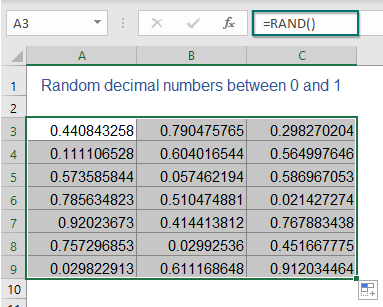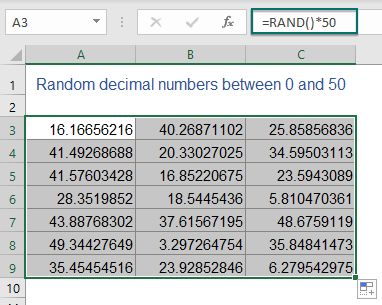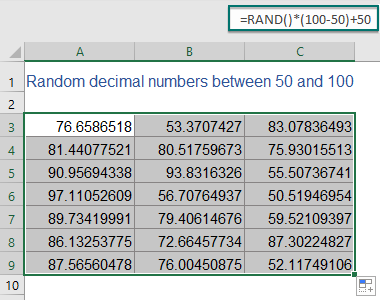公式 描述 =INT(兰德()*N) 生成 0 到 N 之间的随机整数。 =INT(兰德()*(BA)+A) 在您指定的任意两个数字之间生成随机整数。 (A 是下界值和 B 是上限值。）

 =INT(兰德()*100) =INT(兰德()*(500-200)+200)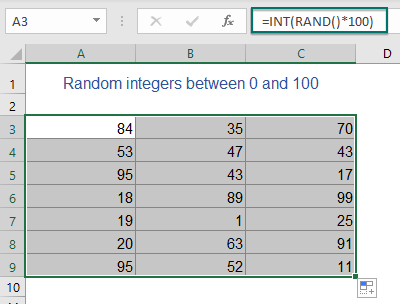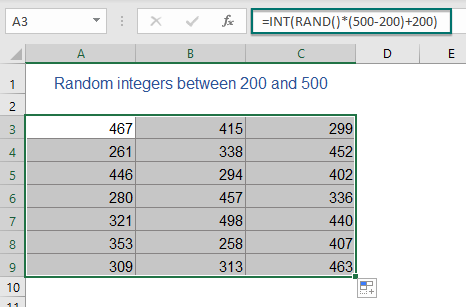###### RANDBETWEEN 函数生成随机数

=RANDBETWEEN(bottom, top)
• 底部, 最佳：你想得到的随机数范围的最低和最高数字。

=RANDBETWEEN(100, 200)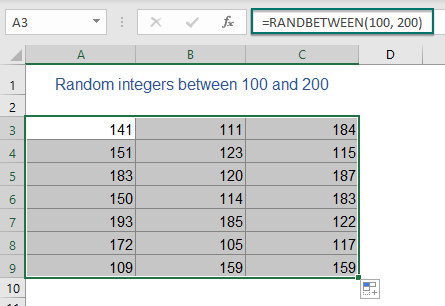=RANDBETWEEN(-100, 100)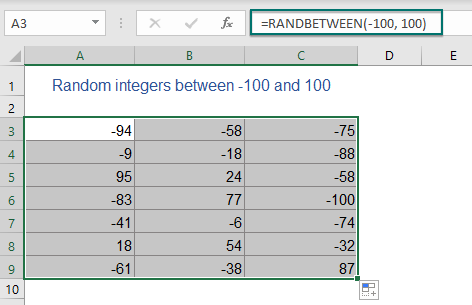• 带一位小数的随机数： =随机数（底部*10，顶部*10）/10
• 两位小数的随机数： =随机数（底部*100，顶部*100）/100
• 三位小数的随机数： =随机数（底部*1000，顶部*1000）/1000
• ...

=RANDBETWEEN(10*100, 50*100)/100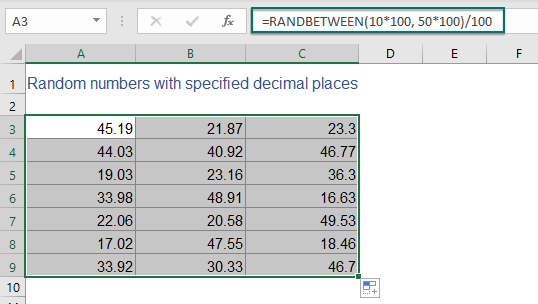###### 用户定义函数在两个值之间生成随机数

1。 按住 ALT + F11 键打开 Microsoft Visual Basic应用程序 窗口。

2。 点击 插页 > 模块，然后将以下代码粘贴到 模块 窗口。

VBA 代码：生成随机数

``````Public Function RandomNumbers(Num1 As Long, Num2 As Long, Optional Decimals As Integer)
'Updateby Extendoffice
Application.Volatile
Randomize
If IsMissing(Decimals) Or Decimals = 0 Then
RandomNumbers = Int((Num2 + 1 - Num1) * Rnd + Num1)
Else
RandomNumbers = Round((Num2 - Num1) * Rnd + Num1, Decimals)
End If
End Function
``````

3. 然后，关闭代码并返回工作表，在空白单元格中键入此公式 = RandomNumbers（X，Y，Z）.

1.) 要生成 50 到 200 之间的随机整数，请使用以下公式：

=RandomNumbers(50,200,0)

2.) 要插入 50 到 200 之间带有 2 个小数位的随机数，请应用以下公式：

=RandomNumbers(50,200,2)

4. 最后，根据需要将公式拖动并复制到其他单元格，您将获得如下屏幕截图所示的结果：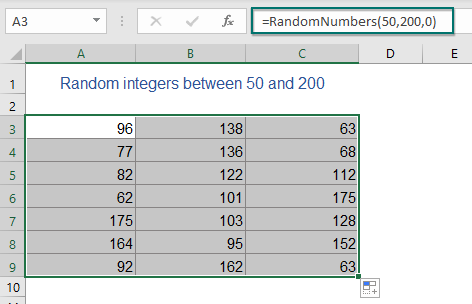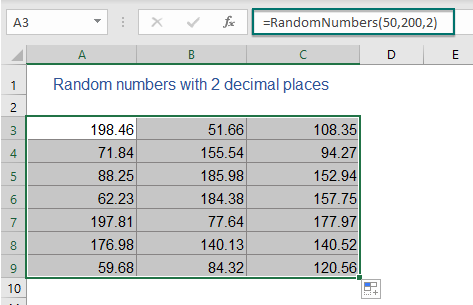###### 在两个数字之间生成随机数的便捷功能

1. 选择要在其中生成随机数的单元格范围，然后单击 库工具 > 插页 > 插入随机数据，请参见屏幕截图：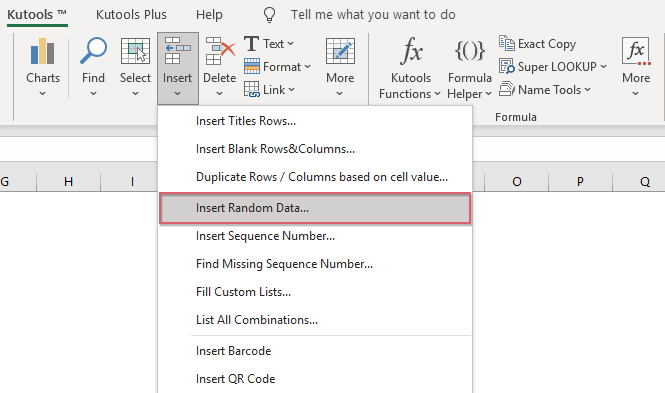2。 在 插入随机数据 对话框：

1.) 生成随机整数：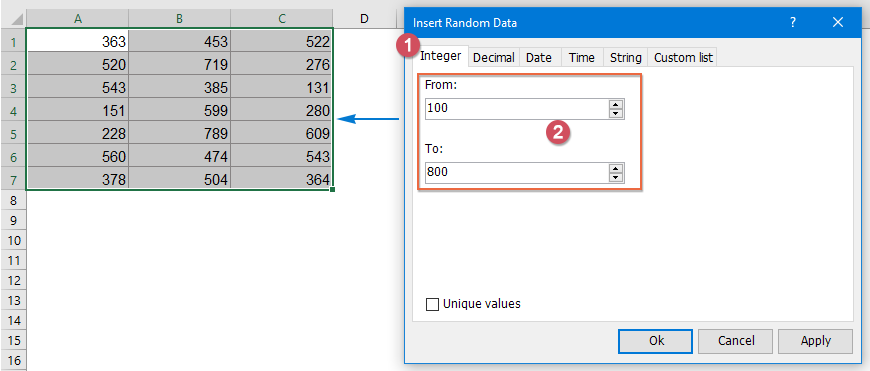2.) 生成具有特定小数位的随机数：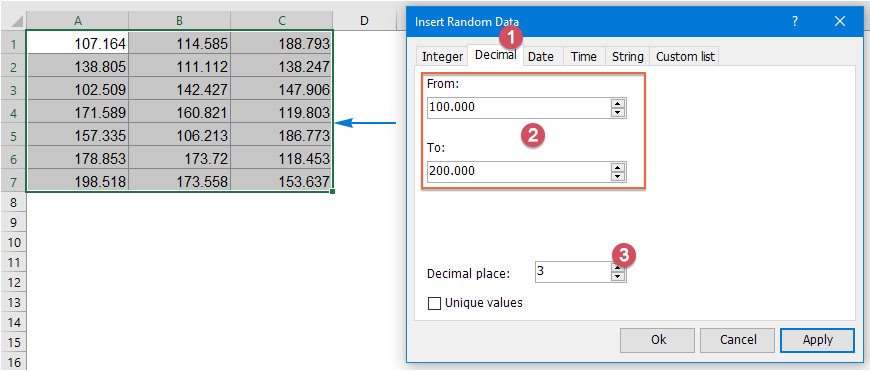#### 1.2 生成不重复的随机数（唯一随机数）

###### 使用数组公式生成不重复的随机数

1. 在两个单元格中指定下限和上限值。 在这个例子中，我将在单元格 B100 和 B200 中输入 2 和 3，看截图：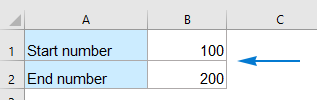2. 然后，将以下公式复制到一个空白单元格中，例如D3，（不要将公式放入第一行的单元格中），然后按 Ctrl+Shift+Enter 键一起得到第一个数字，看截图：

=IFERROR(LARGE(ROW(INDIRECT(\$B\$1&":"&\$B\$2))*NOT(COUNTIF(\$D\$2:D2,ROW(INDIRECT(\$B\$1&":"&\$B\$2)))),RANDBETWEEN(1,\$B\$2-\$B\$1-ROW(A1)+2)),"")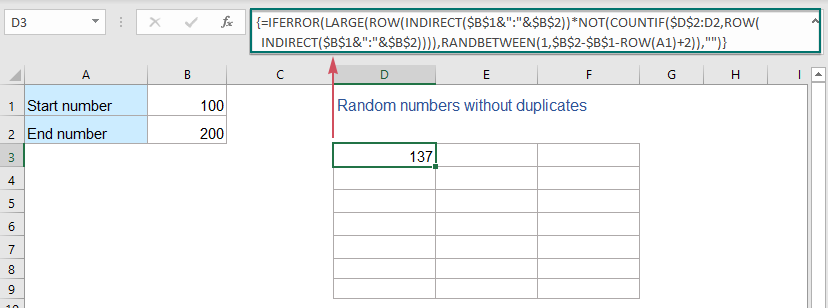3. 然后，将此公式拖动并复制到其他单元格，因为您要生成 100 到 200 之间的随机数：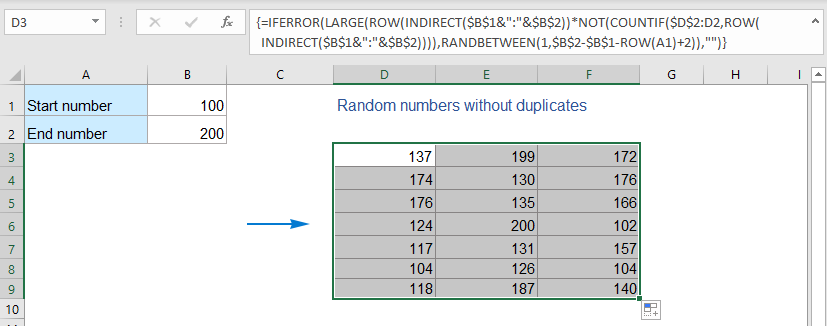###### 使用VBA代码生成没有重复的随机数

1。 按住 ALT + F11 键打开 Microsoft Visual Basic应用程序 窗口。

2。 点击 插页 > 模块，然后将以下代码粘贴到 模块 窗口。

VBA代码：生成没有重复的随机数

``````Sub Range_RandomNumber()
'Updateby Extendoffice
Dim xStrRange As String
Dim xRg, xCell, xRg1 As Range
Dim xArs As Areas
Dim xNum_Lowerbound As Integer
Dim xNum_Upperbound  As Integer
Dim xI, xJ, xS, xR As Integer
xStrRange = "A1:B20"
xNum_Lowerbound = 100
xNum_Upperbound = 200
Set xRg = Range(xStrRange)
Set xArs = xRg.Areas
xRgCount = 0
For xI = 1 To xArs.Count
Set xCell = xArs.Item(xI)
xRgCount = xCell.Count + xRgCount
Next xI
xS = (xNum_Upperbound - xNum_Lowerbound + 1)
If xRgCount > xS Then
MsgBox ("Number of cells greater than the number of unique random numbers!")
Exit Sub
End If
xRg.Clear
For xI = 1 To xArs.Count
Set xCell = xArs.Item(xI)
For xJ = 1 To xCell.Count
Set xRg1 = xCell.Item(xJ)
xR = Int(xS * Rnd + xNum_Lowerbound)
Do While Application.WorksheetFunction.CountIf(xRg, xR) >= 1
xR = Int(xS * Rnd + xNum_Lowerbound)
Loop
xRg1.Value = xR
Next
Next
End Sub
``````

3。 然后按 F5 键运行此代码，唯一的随机数将被插入到指定的范围内。

###### 生成具有强大功能的无重复随机数

1. 选择要在其中生成随机数的单元格范围。

2。 然后点击 库工具 > 插页 > 插入随机数据. 在弹出的对话框中，请进行以下操作：

• 在下面 整数 标签，在 框中，输入您将在其间生成随机数的数字范围；
• 访问 独特价值 选项;
• 然后点击 Ok or 申请 按钮获取唯一的随机数，如下图所示。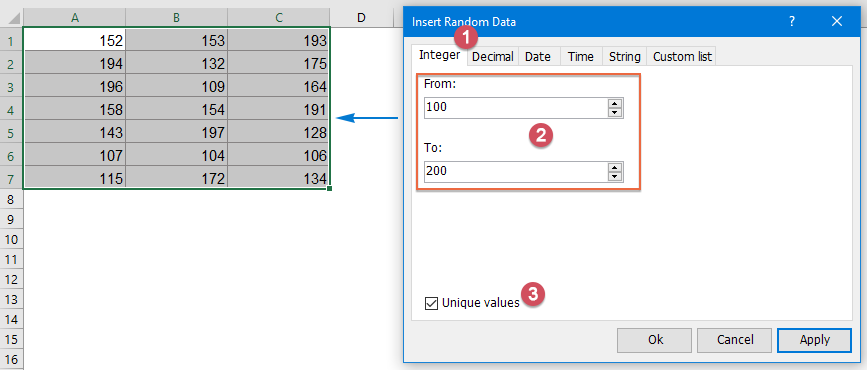#### 1.3 Excel中生成随机偶数或奇数

 公式 描述 =EVEN(RANDBETWEEN(底部，顶部)) 在两个给定数字之间生成随机偶数。 =奇数（随机之间（底部，顶部）） 在两个给定数字之间生成随机奇数。

=EVEN(RANDBETWEEN(10,100))             (Generate random even numbers)
=ODD(RANDBETWEEN(10,100))
(Generate random odd numbers)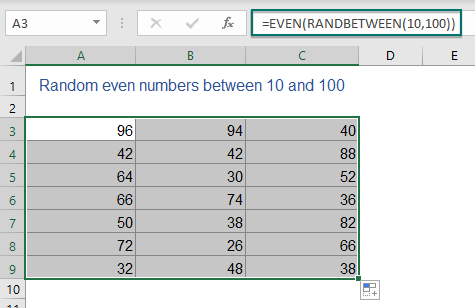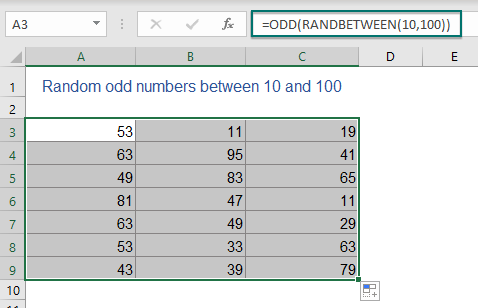#### 1.4 生成加起来为指定值的随机数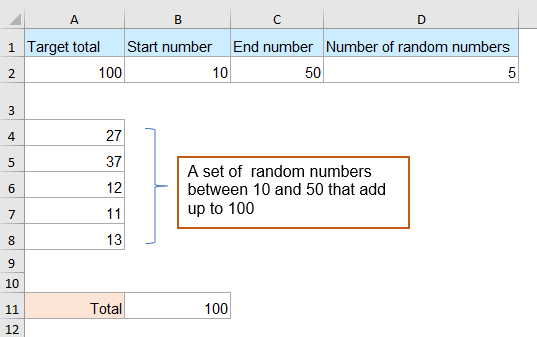###### 使用公式生成与特定值相加的随机数

1. 首先，您应该创建您需要的数据：预定的总值、开始编号、结束编号以及您想要生成的随机数数量，如下图所示：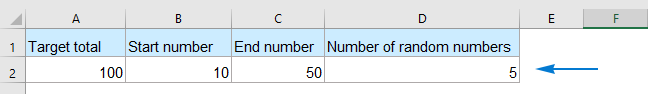2. 然后，请将以下公式复制到要生成数字的空白单元格中。 在本例中，我将公式放入单元格 A4，然后按 输入 获取第一个随机数的键，见截图：

=RANDBETWEEN(MAX(\$B\$2,\$A\$2-((\$D\$2-ROWS(\$A\$4:\$A4))*\$C\$2)),MIN(\$C\$2,\$A\$2-((\$D\$2-ROWS(\$A\$4:\$A4))*\$B\$2)))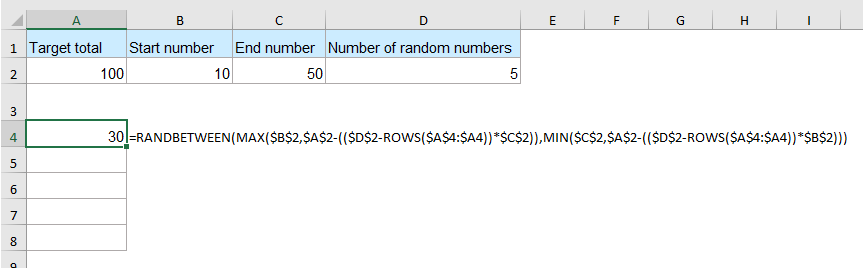3. 继续将以下公式复制到A5单元格中，然后按 输入 获取第二个随机数的键，见截图：

=IF(ROW()=\$D\$2+3,\$A\$2-SUM(\$A\$4:\$A4),IF(ROW()>\$D\$2+3,"",RANDBETWEEN(MAX(\$B\$2,\$A\$2-(SUM(\$A\$4:\$A4)+(\$D\$2-ROWS(\$A\$4:\$A5))*\$C\$2)),MIN(\$C\$2,\$A\$2-(SUM(\$A\$4:\$A4)+(\$D\$2-ROWS(\$A\$4:\$A5))*\$B\$2)))))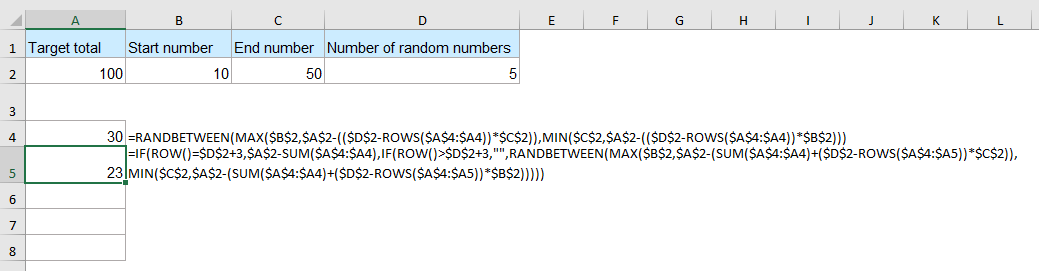4. 然后，选择第二个生成的数字，向下拖动以将此公式复制到下面的三个单元格中。 现在，您将获得 5 个随机数，如下图所示：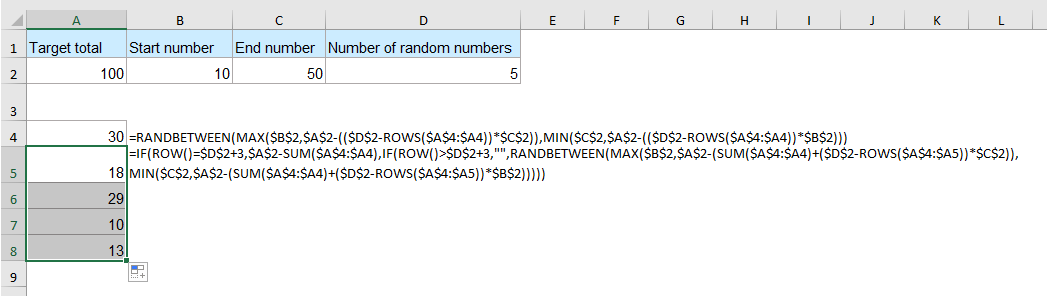5. 为了测试结果，您可以将这些数字相加以检查总和是否为100，您可以按F9动态刷新随机数，它们的总和始终为100。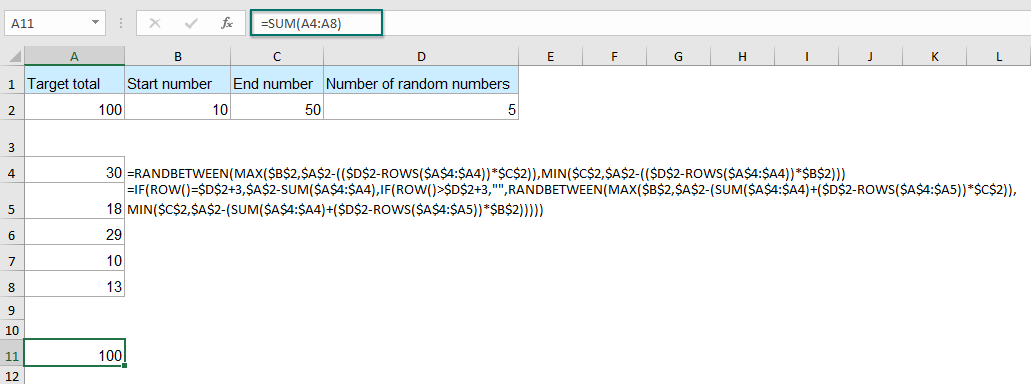###### 生成具有惊人功能的随机数组合，这些组合加起来为特定值

1. 首先，您应该列出您指定的数字。 在这里，我们列出了 10 到 50 之间的所有数字，如下图所示：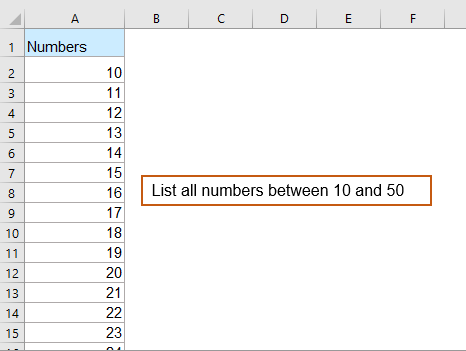2。 然后，点击 库工具 > 内容 > 组成一个数字，请参见屏幕截图：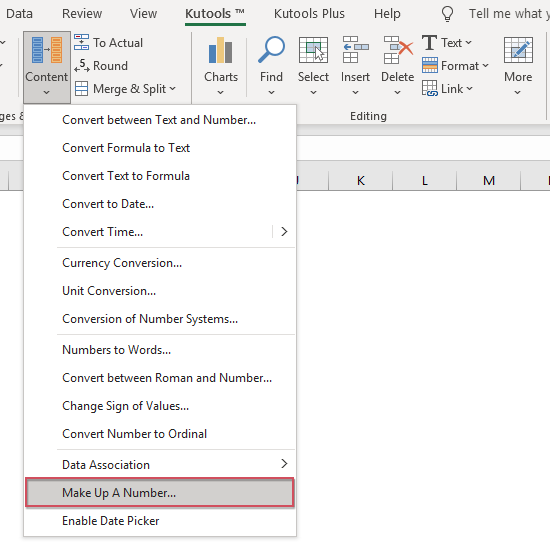3。 在弹出的 补数 对话框，请执行以下操作：

• 数据源 在方框中，选择数字列表以查找哪些数字加起来为100；
• 在下面 附加选项, 在 Sum 文本框中输入总值。 我们在这里输入 100 进入文本框；
• 单向阀 保存在新表中 选项，如果您想在新工作表中列出结果；
• 点击 OK 按钮。 看截图：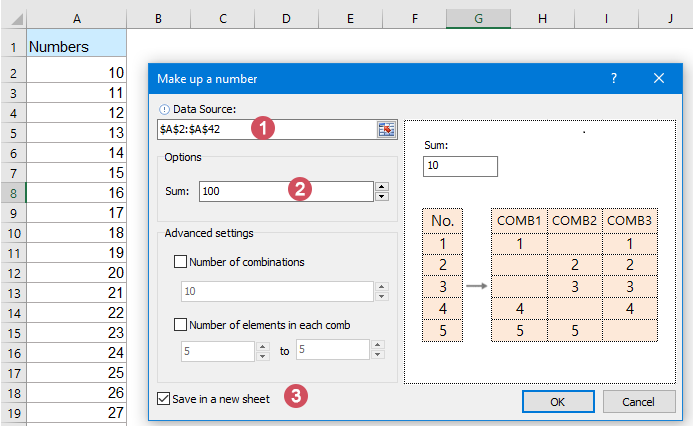4. 处理后，您将看到所有总和为 100 的随机数集合，其中包含 10 到 50 的数字，如下所示。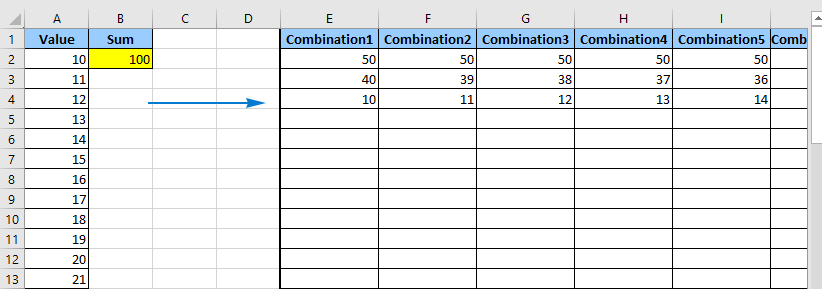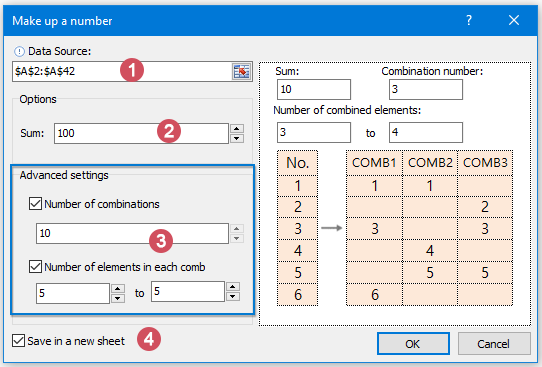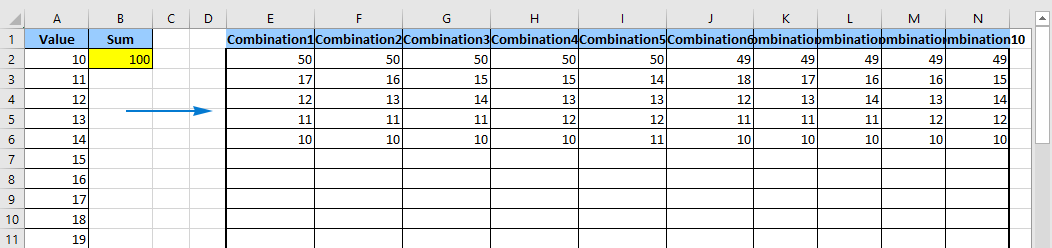#### 1.5 用公式生成随机字母和文本串

###### 用公式生成随机字母和文本字符串

 公式 描述 =CHAR(RANDBETWEEN(65, 90)) 在 A 和 Z 之间生成随机大写字母。 =CHAR(RANDBETWEEN(97, 122)) 在 a 和 z 之间生成随机小写字母。 =CHAR(RANDBETWEEN(33, 47)) 生成随机的特殊字符，例如：! " # \$ % & ' ( ) * + , - ./

 =CHAR(RANDBETWEEN(65, 90)) =CHAR(RANDBETWEEN(97, 122)) =CHAR(RANDBETWEEN(33, 47))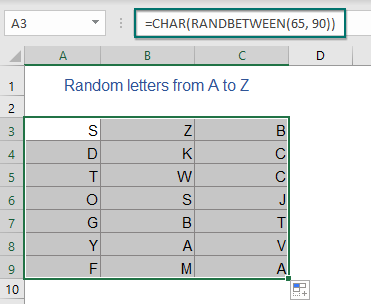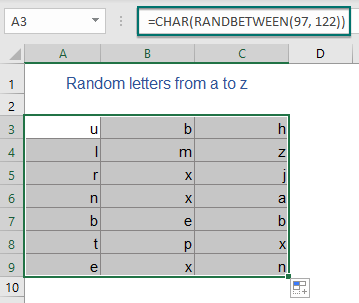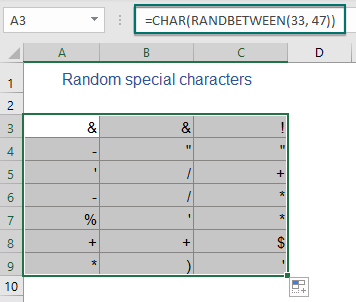1.) 要生成具有四个大写字母的随机字符串，请应用以下公式：

=CHAR(RANDBETWEEN(65,90))& CHAR(RANDBETWEEN(65,90)) & CHAR(RANDBETWEEN(65,90)) & CHAR(RANDBETWEEN(65,90))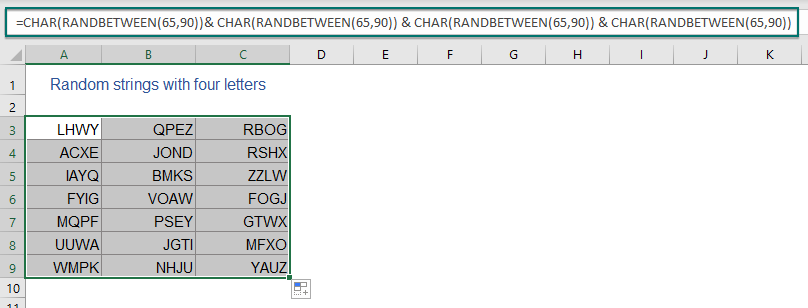2.) 要生成四个小写字母的随机字符串，请应用以下公式：

=CHAR(RANDBETWEEN(97,122))& CHAR(RANDBETWEEN(97,122)) & CHAR(RANDBETWEEN(97,122)) & CHAR(RANDBETWEEN(97,122))

3.) 要生成前两个大写字母和后两个小写字母的随机字符串，请使用以下公式：

=CHAR(RANDBETWEEN(65,90))& CHAR(RANDBETWEEN(65,90)) & CHAR(RANDBETWEEN(97,122)) & CHAR(RANDBETWEEN(97,122))

###### 使用方便的功能生成随机字母和文本字符串

1. 选择一系列单元格以插入字母或字符串。

2。 然后点击 库工具 > 插页 > 插入随机数据, 在弹出的对话框中进行如下操作：

• 点击 标签;
• 单向阀 AZ or A-Z 或您要插入的两者；
• 然后，在 弦长 文本框;
• 最后点击 Ok or 申请 插入字符串，如下图所示。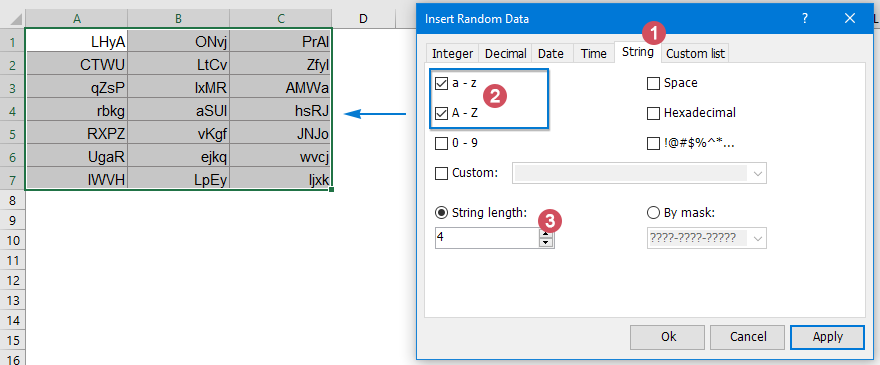#### 1.6 Excel中生成带有字母数字字符的随机密码

###### 使用公式生成带有字母数字字符的随机密码

=CHAR(RANDBETWEEN(65,90))&CHAR(RANDBETWEEN(97,122))&CHAR(RANDBETWEEN(97,122))&CHAR(RANDBETWEEN(65,90))&RANDBETWEEN(100,999)&CHAR(RANDBETWEEN(33,47))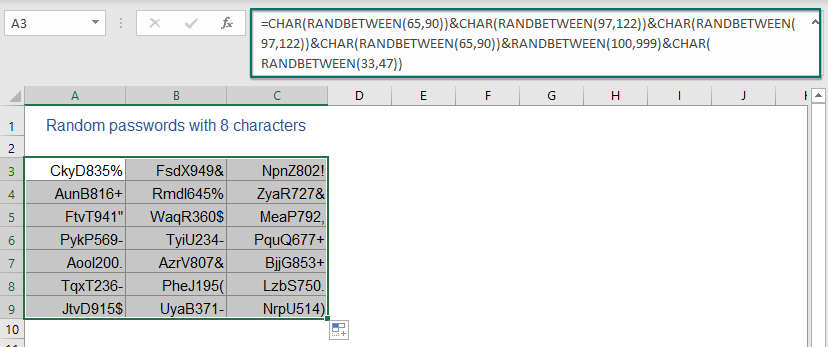###### 使用用户定义函数生成带有字母数字字符的随机密码

1。 按住 ALT + F11 键，然后打开 Microsoft Visual Basic应用程序 窗口。

2。 点击 插页 > 模块，然后将以下宏粘贴到 模块 窗口。

VBA 代码：在 Excel 中生成随机密码

``````Function RandomizeF(Num1 As Integer, Num2 As Integer)
'Updateby Extendoffice
Dim Rand As String
Application.Volatile
getLen = Int((Num2 + 1 - Num1) * Rnd + Num1)
Do
i = i + 1
Randomize
Rand = Rand & Chr(Int((85) * Rnd + 38))
Loop Until i = getLen
RandomizeF = Rand
End Function
``````

3. 然后关闭代码并返回到工作表。 在单元格中输入此公式 = RandomizeF（8,10） 生成最小长度为 8 个字符，最大长度为 10 个字符的随机文本字符串。

4. 然后根据需要将公式拖动并复制到其他单元格。 将创建长度在 8 到 10 之间的带有字母数字和特定字符的随机字符串。 看截图：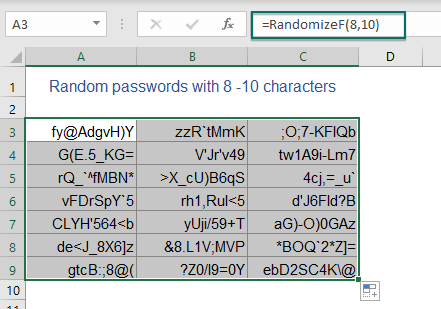###### 使用简单的功能生成带有字母数字字符的随机密码

1. 选择要插入密码的单元格范围。

2。 然后点击 库工具 > 插页 > 插入随机数据. 在弹出的对话框中，请进行以下操作：

• 点击 标签;
• 根据需要检查字符类型；
• 然后，在 弦长 文本框;
• 最后点击 Ok or 申请 生成密码，如下图所示。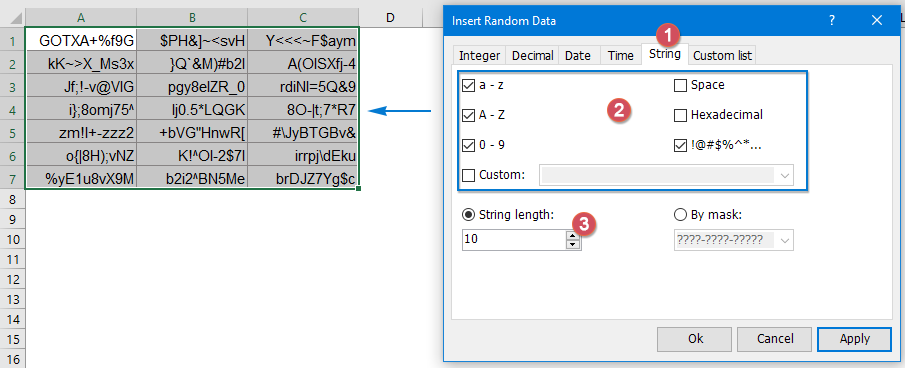#### 1.7 在Excel中生成随机特定文本

###### 使用公式生成随机特定文本

=CHOOSE(RANDBETWEEN(1,n),"Value_1","Value_2","Value_3",…"Value_n")
• 值_1、值_2、值_3、值_n ：代表你想随机列出的文本值；
• n ：要使用的文本值的数量。

=CHOOSE(RANDBETWEEN(1,5),"Chemistry","Physics","Geography","Biology","Economics")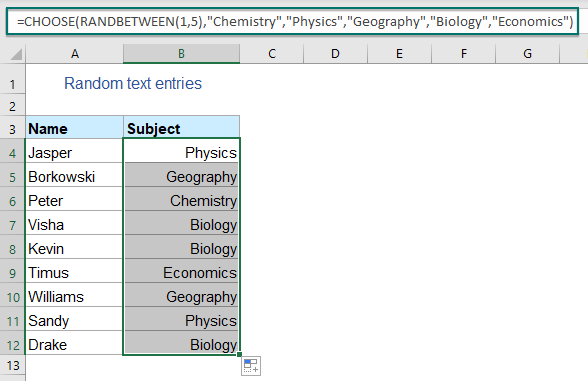###### 使用快速方法生成随机特定文本

1. 选择要插入特定文本的单元格范围。

2。 然后点击 库工具 > 插页 > 插入随机数据, 在弹出的对话框中进行如下操作：

• 点击 自订清单 标签;
• 然后，单击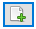按钮打开另一个 Kutools for Excel 提示框中，输入或选择您要随机列出的自定义文本值。 （手动输入时，条目应以逗号分隔。）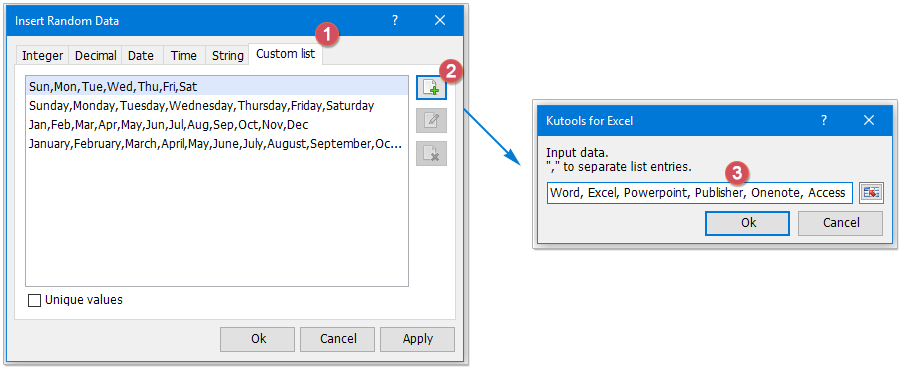3。 然后，点击 Ok 回到了 插入随机数据 对话框中，您自己的自定义文本列表已显示在列表框中。 现在，选择新的列表项，单击 Ok or 申请 按钮将值随机插入选定的单元格。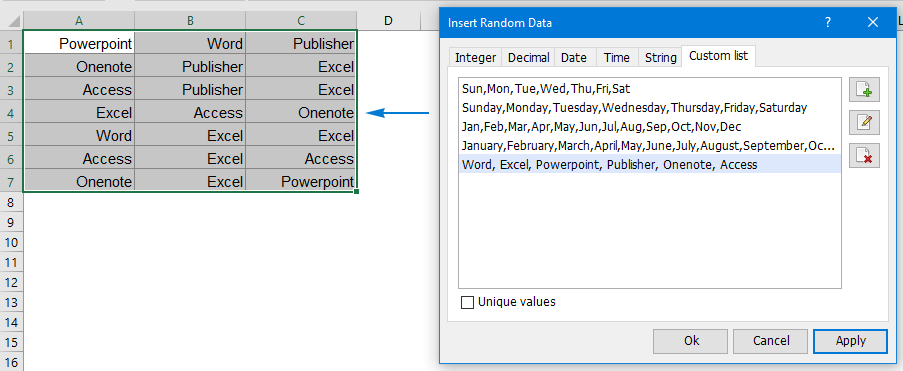#### 1.8 从 Excel 列表中生成或选择随机值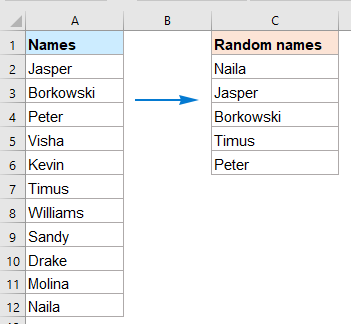###### 使用 INDEX、RANDBETWEEN 和 ROWS 函数从列表中生成随机值

1. 请将以下公式复制到要放置提取值的空白单元格中：

=INDEX(\$A\$2:\$A\$12,RANDBETWEEN(1,ROWS(\$A\$2:\$A\$12)),1)

2. 然后，将填充手柄向下拖动到要显示随机值的几个单元格，您将获得如下屏幕截图所示的结果：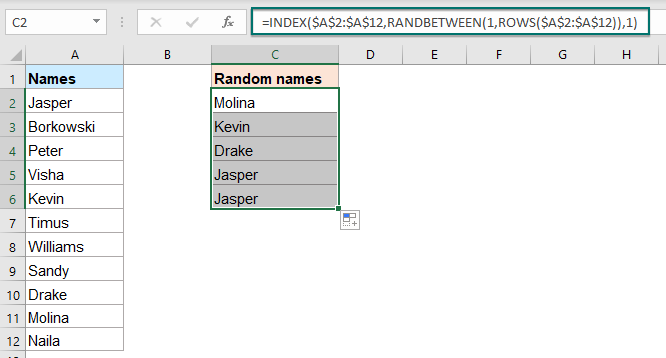###### 使用 INDEX、RANK.EQ 函数从没有重复项的列表中生成随机值

1. 在空白单元格中输入以下公式以获取随机数列表，请参见屏幕截图：

=RAND()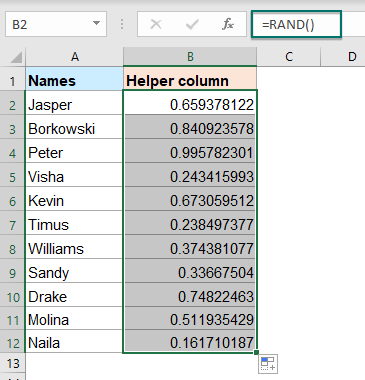2. 然后，将以下公式复制到要提取一些随机值的另一列的单元格中，然后将此公式拖动并复制到以下单元格以显示一些非重复随机值，请参见屏幕截图：

=INDEX(\$A\$2:\$A\$12,RANK.EQ(\$B2,\$B\$2:\$B\$12))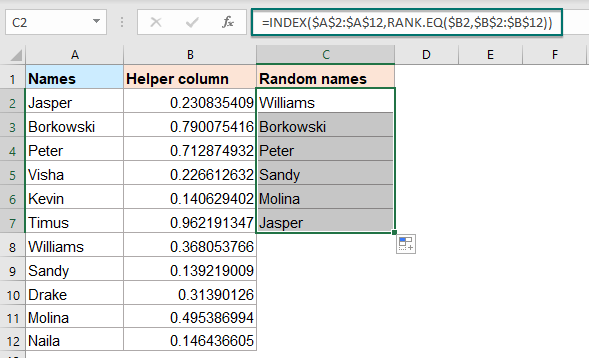###### 从具有出色功能的范围中选择随机单元格、行、列

1. 选择要从中选择一些随机值的单元格列表。

2。 然后，单击 库工具 > Range > 随机排序/选择范围，请参见屏幕截图：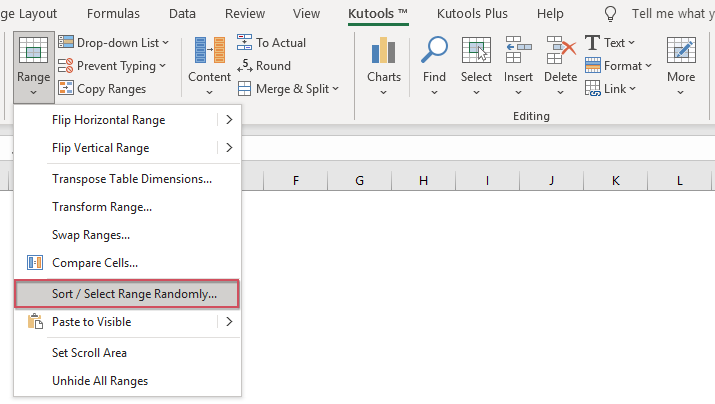3。 在 随机排序/选择范围 对话框，请执行以下操作：

• 单击 选择 标签;
• 然后，在框中输入要随机选择的单元格数 细胞数 选择 框;
• 选择类型 部分，根据需要选择一项操作。 在这种情况下，我会选择 选择随机单元格 选项。
• 然后，单击 Ok or 申请 按钮，将一次随机选择五个单元格，见截图：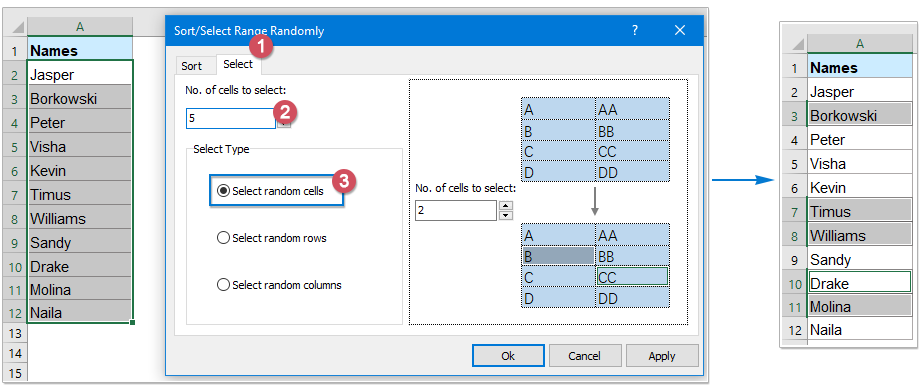4. 选择单元格后，您可以根据需要将它们复制并粘贴到其他单元格。

#### 1.9 Excel中将数据随机分组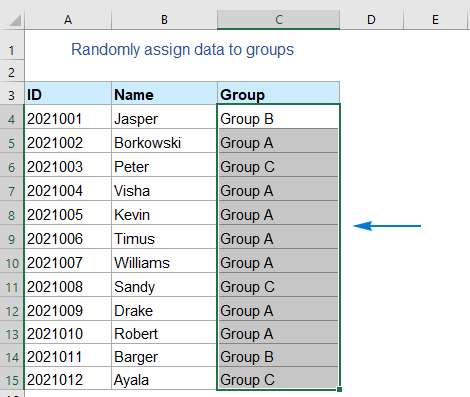###### 使用公式将数据随机分组

1. 请将以下公式复制或输入到要生成组的单元格中：

=CHOOSE(RANDBETWEEN(1,3),"Group A","Group B","Group C")

2. 然后，拖动填充柄将此公式填充到其他单元格，名称将分为三组，如下图所示：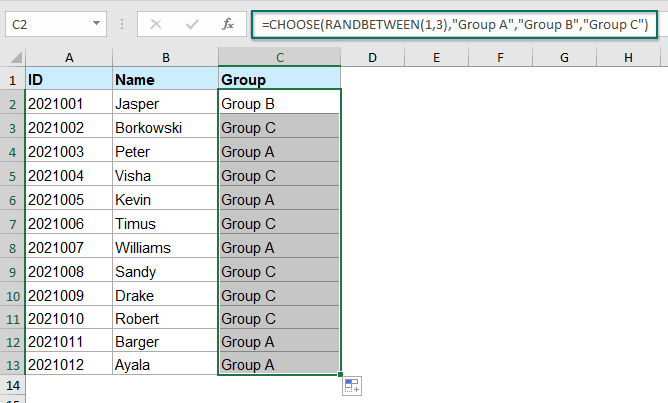###### 使用公式将数据随机分配到相同数量的组

1。 输入以下公式： = RAND（） 进入一个空白单元格以获取随机数列表，请参见屏幕截图：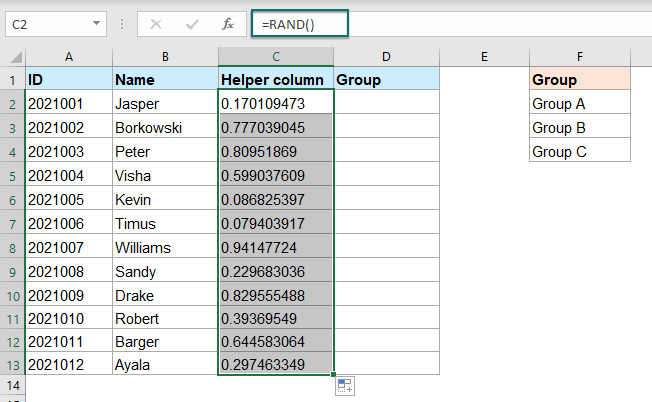2. 然后，在下一列中，例如，在单元格 D2 中，复制或键入以下公式：

=INDEX(\$F\$2:\$F\$4, ROUNDUP(RANK(C2,\$C\$2:\$C\$13)/4,0))

3. 向下拖动填充手柄为数据列表生成随机组，名称将被分成相等的组，见截图：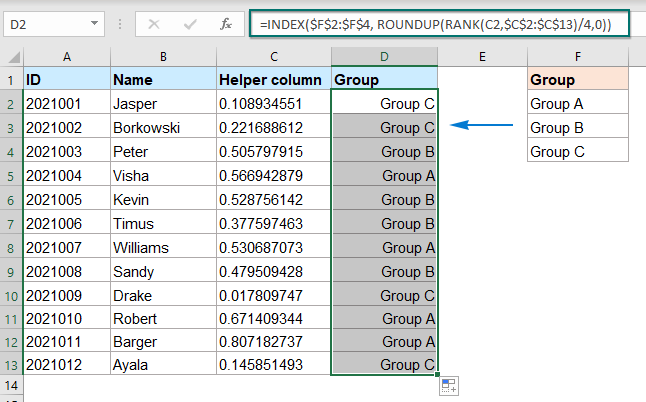#### 1.10 Excel中生成随机日期

###### 使用公式在两个给定日期之间生成随机日期

1. 选择要插入随机日期的单元格，然后输入以下公式：

=RANDBETWEEN(DATE(2021, 5, 1),DATE(2021, 10, 15))

2. 然后，将此公式拖动并复制到要填充此公式的其他单元格，单元格中将显示五位数，如下图所示：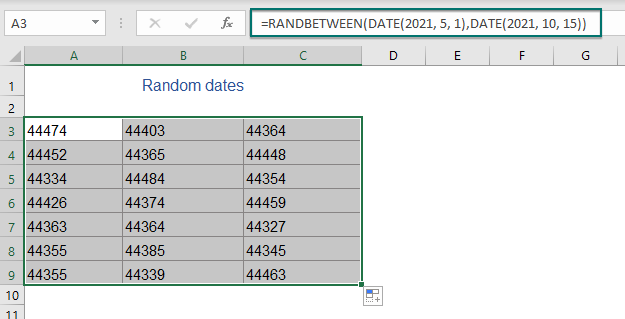3. 然后，您应该将数字格式化为日期格式。 请选择公式单元格，然后右键单击，选择 单元格格式 从上下文菜单。

4。 在 单元格格式 对话框，单击 选项卡，然后选择 Date 来自 类别 窗格，然后从 类型 下拉列表。 看截图：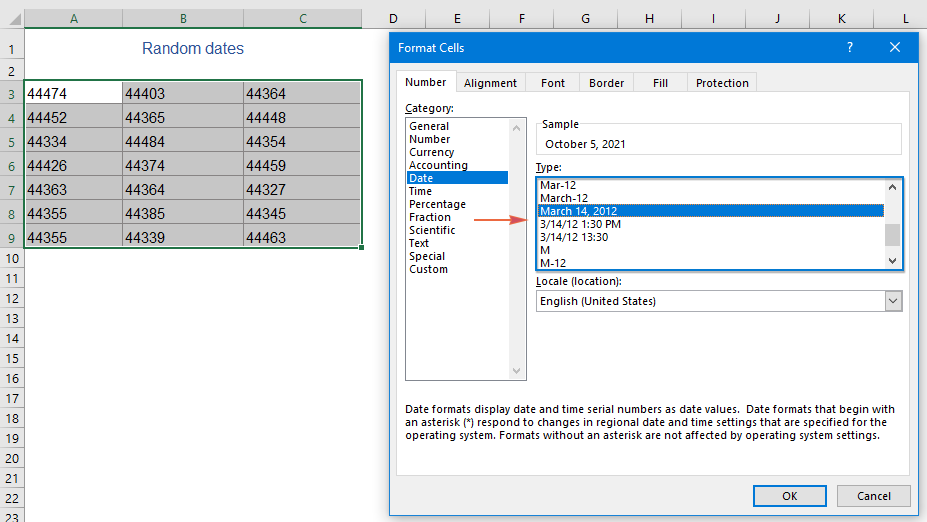5。 点击 OK 关闭对话框。 现在，数字已转换为正常日期。 看截图：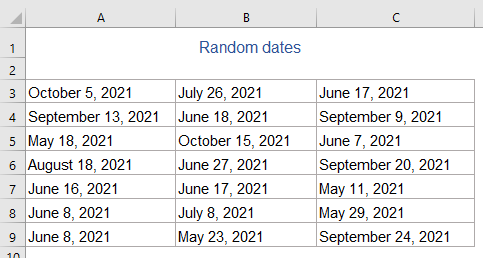=WORKDAY(RANDBETWEEN(DATE(2021, 5, 1),DATE(2021, 10, 15))-1,1)

###### 使用惊人的功能在两个给定日期之间生成随机日期

Kutools for Excel插入随机数据 还提供了一个选项来帮助您在两个给定日期之间生成随机日期、工作日、周末。

1. 选择要插入随机日期的单元格范围。

2。 然后点击 库工具 > 插页 > 插入随机数据, 在弹出的对话框中进行如下操作：

• 点击 Date 标签;
• 然后，指定日期范围。 在这个例子中，我将选择 5/1/202110/15/2021.
• 然后，选择日期类型 - 工作日 日期， 周末 根据您的需要日期或两者。
• 最后点击 Ok or 申请 如下图所示随机生成日期。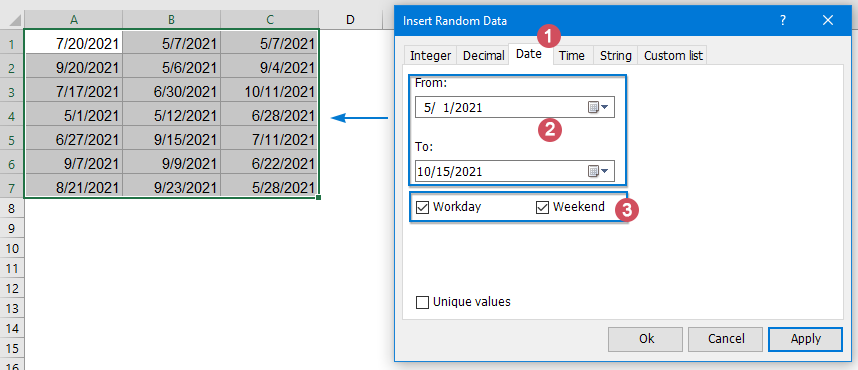#### 1.11 在Excel中生成随机时间

###### 用公式生成随机时间

=TEXT(RAND(),"HH:MM:SS")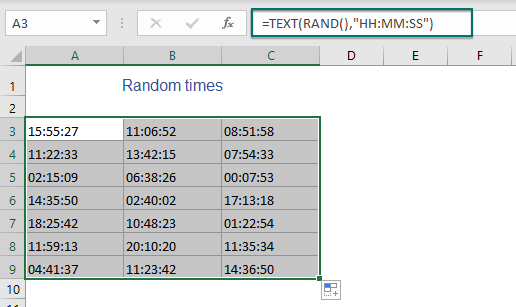=TEXT(RAND()*(18-10)/24+10/24,”HH:MM:SS”)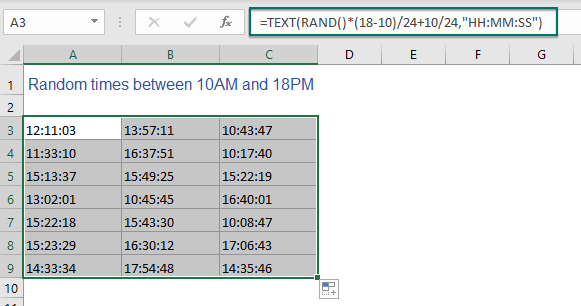=TEXT(FLOOR(RAND(),"0:15"),"HH:MM:SS")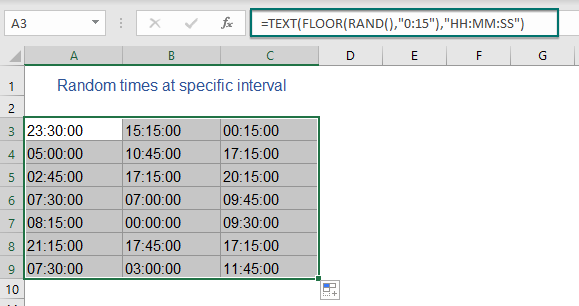###### 使用方便的功能在两个给定时间之间生成随机时间

1. 选择生成时间的单元格范围。

2. 然后点击 库工具 > 插页 > 插入随机数据, 在弹出的对话框中进行如下操作：

• 点击 时间 标签;
• 然后，指定时间范围。 在这个例子中，我将选择 中午 9:00 点下午 16：30 点.
• 最后点击 Ok or 申请 生成随机时间，如下图所示。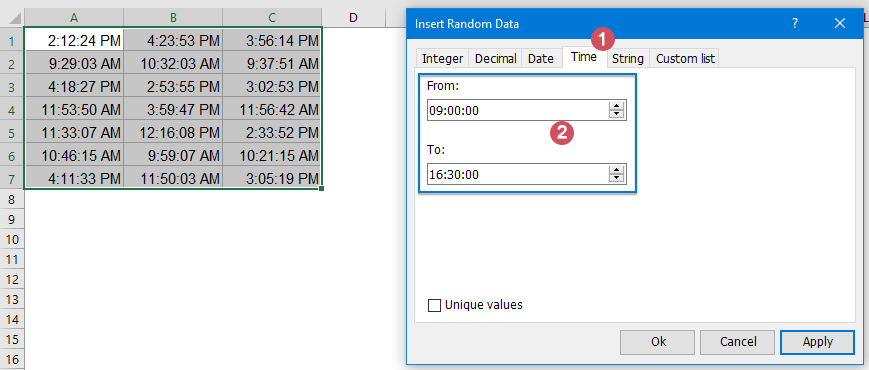###### 使用公式在两个日期时间之间生成随机日期和时间

1. 将以下公式输入或复制到要生成随机日期时间的单元格中：

=TEXT(RAND()*("2021-10-15 12:00:00"-"2021-1-1 9:00")+"2021-1-1 9:00:00","YYYY-MM-DD HH:MM:SS")

2. 然后，将此公式拖动并复制到您希望显示随机日期时间的其他单元格，请参阅屏幕截图：### 在 Excel 365 / 2021 中生成随机数、文本、日期

RANDARRAY 函数用于返回您指定的任意两个数字之间的随机数数组。

RANDARRAY 函数的语法是：

=RANDARRAY([rows],[columns],[min],[max],[integer])
• （可选）：要返回的随机数的行数； （如果省略，默认=1）
• （可选）：要返回的随机数列数； （如果省略，默认=1）
• 分钟 （可选）：要返回的最小数量； （如果省略，默认=0）
• 最大 （可选）：要返回的最大数量； （如果省略，默认=1）
• 整数 （可选）：返回整数或十进制值。 TRUE 表示整数，False 表示十进制数。 （如果省略，默认=FALSE）

• 1. RANDARRAY 函数有五个参数，都是可选的，如果没有指定参数，RANDARRAY 将返回一个 0 到 1 之间的十进制值。
• 2. 如果行或列参数是十进制数，它们将被截断为小数点前的整数（例如 3.9 将被视为 3）。
• 3.最小数必须小于最大数，否则返回#VALUE！ 错误。
• 4.这个RANDARRAY返回一个数组，当RANDARRAY在一个工作表中返回多个结果时，结果会溢出到相邻的单元格中。

#### 2.1 在 Excel 365 / 2021 中生成随机数

###### 用公式在两个数字之间生成随机数

=RANDARRAY(6, 4, 50, 200, TRUE)               (Generate random integers between 50 and 200)
=RANDARRAY(6, 4, 50, 200, FALSE)
(Generate random decimals between 50 and 200)

• 6：表示返回6行随机数；
• 4：表示返回4列随机数；
• 50, 200：要在其间生成数字的最小值和最大值；
• TRUE: 表示返回整数；
• FALSE: 表示返回十进制数。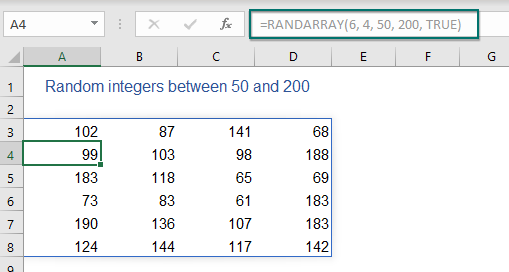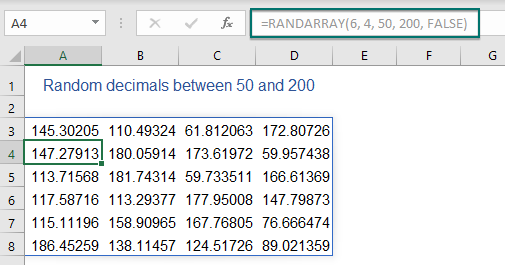###### 用公式生成不重复的随机数

=INDEX(UNIQUE(RANDARRAY(n^2, 1, min, max, TRUE)), SEQUENCE(n))

=INDEX(UNIQUE(RANDARRAY(n^2, 1, min, max, FALSE)), SEQUENCE(n))
• n：要生成的值的数量；
• 分钟：最小值；
• 最大: 最大值。

=INDEX(UNIQUE(RANDARRAY(8^2, 1, 50, 100, TRUE)), SEQUENCE(8))          (Unique random integers)
=INDEX(UNIQUE(RANDARRAY(8^2, 1, 50, 100, FALSE)), SEQUENCE(8))
(Unique random decimals)

• 8：表示返回8个随机数；
• 50, 100：要在其间生成数字的最小值和最大值。
• TRUE: 表示返回整数；
• FALSE: 表示返回十进制数。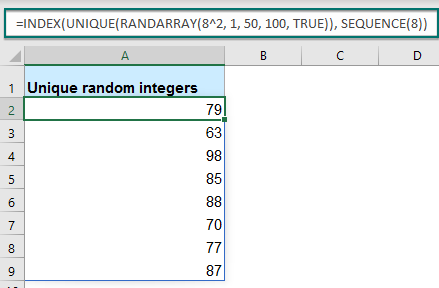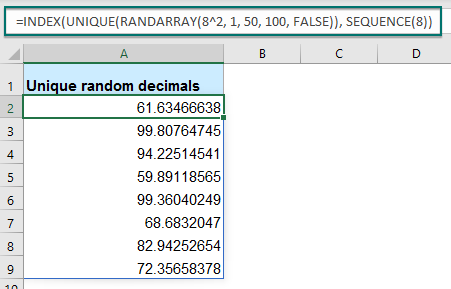=INDEX(UNIQUE(RANDARRAY(n^2, 1, min, max, TRUE)), SEQUENCE(rows, columns))

=INDEX(UNIQUE(RANDARRAY(n^2, 1, min, max, FALSE)), SEQUENCE(rows, columns))
• n: 要插入数字的单元格数，您可以将其提供为行数 * 列数； 例如，要填充 8 行 3 列，请使用 24^2。
• ：要填充的行数；
• : 要填充的列数；
• 分钟：最低值；
• 最大: 最高值。

=INDEX(UNIQUE(RANDARRAY(24^2, 1, 50, 100, TRUE)), SEQUENCE(8,3))          (Unique random integers)
=INDEX(UNIQUE(RANDARRAY(24^2, 1, 50, 100, FALSE)), SEQUENCE(8,3))
(Unique random decimals)

• 24：表示返回24个随机数，8和3的乘积（行*列）；
• 50, 100：要在其间生成数字的最小值和最大值；
• TRUE: 表示返回整数；
• FALSE: 表示返回十进制数。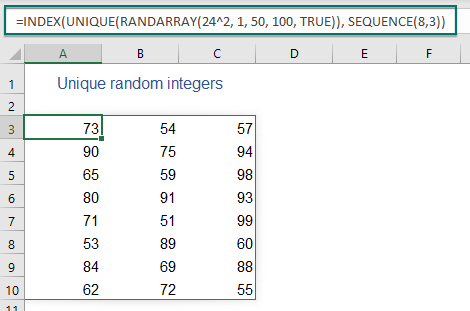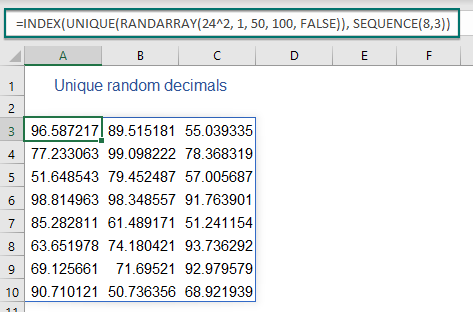#### 2.2 在 Excel 365 / 2021 中生成随机日期

###### 使用公式在两个日期之间生成随机日期

1. 在空白单元格中输入以下公式以生成随机日期，然后按 输入 获取五位数字列表的键，请参见屏幕截图：

=RANDARRAY(10, 1, B1, B2, TRUE)

• 10：表示返回10行随机日期；
• 1：表示返回1列随机日期；
• B1, B2：单元格包含要在其间生成日期的开始日期和结束日期。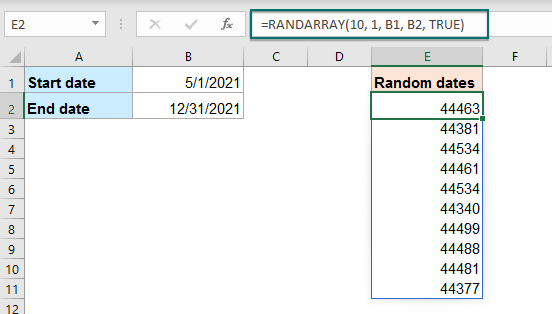2. 然后，您应该将数字格式化为正常日期格式：选择数字，然后右键单击，然后选择 单元格格式 从上下文菜单。 在下面的 单元格格式 对话框，请这样做：

• 单击 标签;
• 然后点击 Date 来自 类别 窗格
• 然后，从 类型 列表框。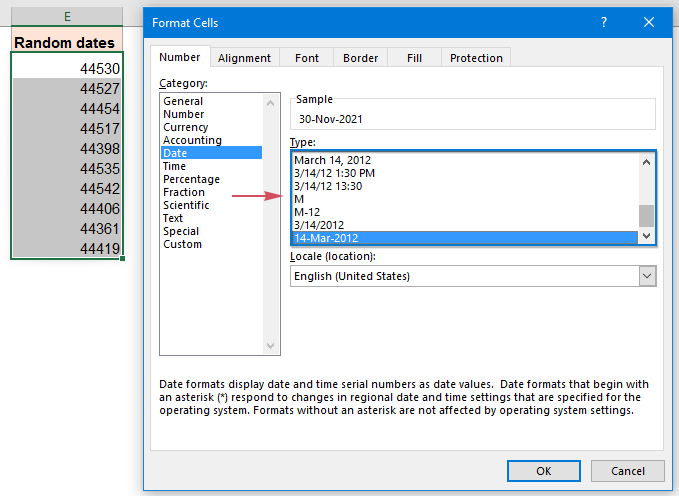3。 然后，单击 OK 按钮，数字将按照您指定的日期格式进行格式化，请参见屏幕截图：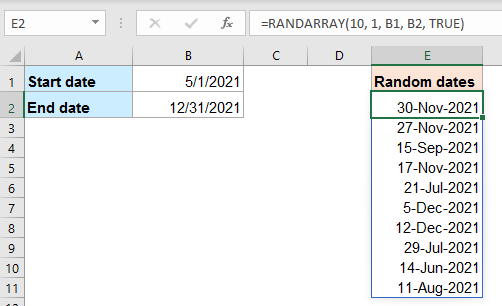=RANDARRAY(10, 1, "5/1/2021", "12/31/2021", TRUE)

###### 使用公式在两个日期之间生成随机工作日

1. 将以下公式输入或复制到空白单元格中，然后按 输入 获取数字列表的键，如下图所示：

=WORKDAY(RANDARRAY(10, 1, B1, B2, TRUE), 1)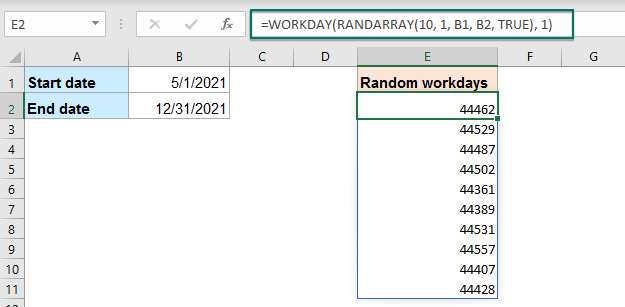2. 然后，根据需要将数字格式化为特定的日期格式 单元格格式 对话框，您将获得正常的日期格式，如下图所示：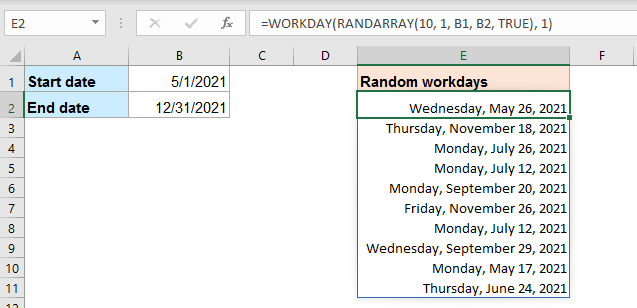=WORKDAY(RANDARRAY(10, 1, "5/1/2021", "12/31/2021", TRUE), 1)

#### 2.3 从 Excel 365 / 2021 中的列表生成或获取随机值

###### 使用公式从列表中生成或获取随机值

=INDEX(data, RANDARRAY(n, 1, 1, ROWS(data), TRUE))
• data：要从中提取随机项的值列表；
• n：您要提取的随机项目的数量。

=INDEX(A2:A12, RANDARRAY(C2, 1, 1, ROWS(A2:A12), TRUE))             （Use a cell reference）
=INDEX(A2:A12, RANDARRAY(3, 1, 1, ROWS(A2:A12), TRUE))
(Type a number directly)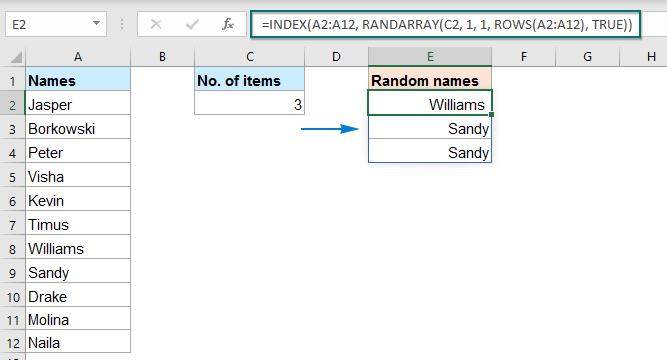###### 使用公式从没有重复项的列表中生成或获取随机值

=INDEX(SORTBY(data, RANDARRAY(ROWS(data))), SEQUENCE(n))
• data：要从中提取随机项的值列表；
• n：您要提取的随机项目的数量。

=INDEX(SORTBY(A2:A12, RANDARRAY(ROWS(A2:A12))), SEQUENCE(C2))             (Use a cell reference)
=INDEX(SORTBY(A2:A12, RANDARRAY(ROWS(A2:A12))), SEQUENCE(5))
(Type a number directly)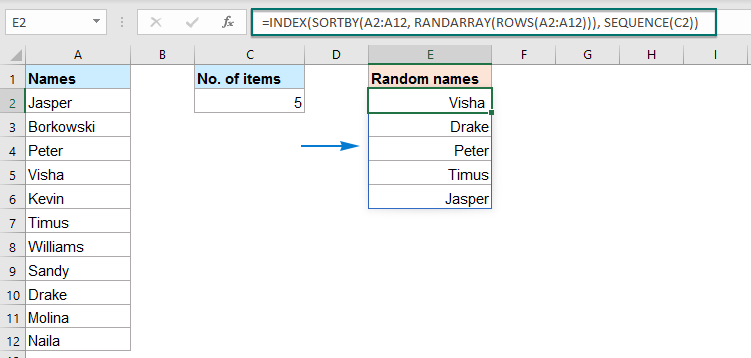#### 2.4 从 Excel 365 / 2021 的范围中生成或选择随机行

###### 使用公式从范围中生成或选择随机行

=INDEX(data, RANDARRAY(n, 1, 1, ROWS(data), TRUE), {1,2,3…})
• data：要从中提取随机行的单元格范围；
• n: 您希望提取的随机行数；
• {1,2,3…}: 要提取的列号。

=INDEX(A2:C12, RANDARRAY(E2, 1, 1, ROWS(A2:C12), TRUE), {1,2,3})               (Use a cell reference)
=INDEX(A2:C12, RANDARRAY(3, 1, 1, ROWS(A2:C12), TRUE), {1,2,3})
(Type a number directly)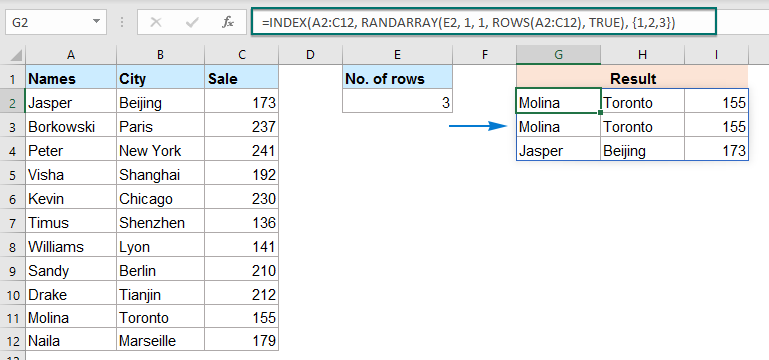###### 使用公式从不重复的范围中生成或选择随机行

=INDEX(SORTBY(data, RANDARRAY(ROWS(data))), SEQUENCE(n), {1,2,3…})
• data：要从中提取随机行的单元格范围；
• n: 您希望提取的随机行数；
• {1,2,3…}: 要提取的列号。

=INDEX(SORTBY(A2:C12, RANDARRAY(ROWS(A2:C12))), SEQUENCE(E2), {1,2,3})            (Use a cell reference)
=INDEX(SORTBY(A2:C12, RANDARRAY(ROWS(A2:C12))), SEQUENCE(5), {1,2,3})
(Type a number directly)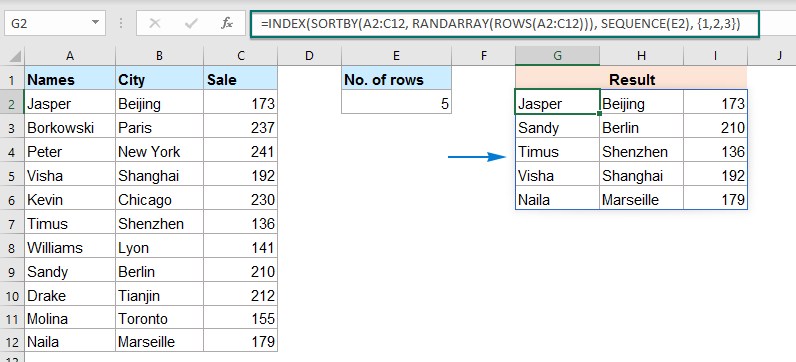### 防止随机结果改变

###### 使用复制和粘贴防止随机结果发生变化

1. 选择带有随机公式的单元格，然后按 按Ctrl + C 复制它们。

2. 然后，右键单击所选范围，然后单击 价值观 选项从 粘贴选项 部分，请参见屏幕截图：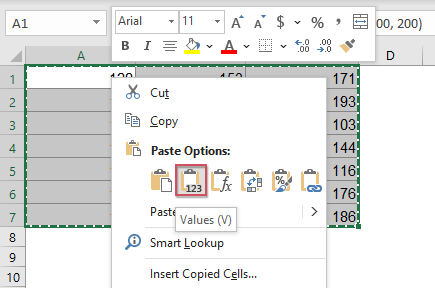3. 并且所有公式单元格都将转换为值，随机值将不再改变。

###### 使用方便的功能防止随机结果发生变化

1. 选择带有随机公式的单元格，然后单击 库工具 > 到实际，请参见屏幕截图：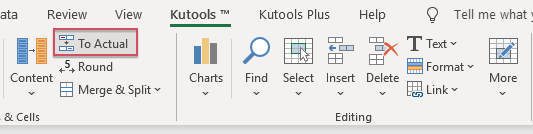2. 现在，所有选定的公式都已转换为值。

## 最佳办公效率工具

### Kutools for Excel解决了您的大多数问题，并使您的生产率提高了80％

• 超级公式栏 （轻松编辑多行文本和公式）； 阅读版式 （轻松读取和编辑大量单元格）； 粘贴到过滤范围...
• 合并单元格/行/列 和保存数据； 拆分单元格内容； 合并重复的行和总和/平均值...防止细胞重复； 比较范围...
• 选择重复或唯一 行; 选择空白行 （所有单元格都是空的）； 超级查找和模糊查找 在许多工作簿中； 随机选择...
• 确切的副本 多个单元格，无需更改公式参考； 自动创建参考 到多张纸； 插入项目符号，复选框等...
• 收藏并快速插入公式，范围，图表和图片； 加密单元 带密码 创建邮件列表 并发送电子邮件...
• 提取文字，添加文本，按位置删除， 删除空间; 创建和打印分页小计； 在单元格内容和注释之间转换...
• 超级滤镜 （将过滤方案保存并应用于其他工作表）； 高级排序 按月/周/日，频率及更多； 特殊过滤器 用粗体，斜体...
• 结合工作簿和工作表; 根据关键列合并表； 将数据分割成多个工作表; 批量转换xls，xlsx和PDF...
• 数据透视表分组依据 周号，周几等 显示未锁定的单元格 用不同的颜色 突出显示具有公式/名称的单元格...### Office选项卡-将选项卡式界面引入Office，使您的工作更加轻松

• 在Word，Excel，PowerPoint中启用选项卡式编辑和阅读，发布者，Access，Visio和Project。
• 在同一窗口的新选项卡中而不是在新窗口中打开并创建多个文档。
• 每天将您的工作效率提高50％，并减少数百次鼠标单击！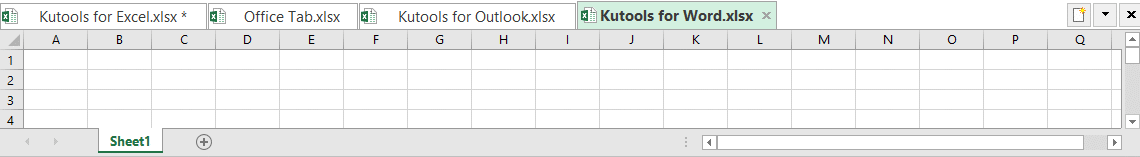0  产品特性